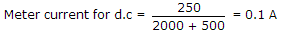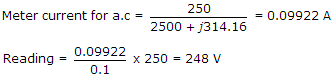# Electronics and Communication Engineering - Measurements and Instrumentation - Discussion

3.

The coil of a moving iron instrument has a resistance of 500 Ω and an inductance of 1 H. It reads 250 V when a 250 V dc is applied. If series resistance is 2000 Ω, its reading when fed by 250 V, 50 Hz ac will be

 [A]. 260 V [B]. 252 V [C]. 250 V [D]. 248 V

Explanation:Suma said: (May 14, 2014) I can't understood the measuring of ac current. Why you put j314.16?

 Annu Kumari said: (Jun 21, 2014) From where you put the value of j314.16?

 Cheetha said: (Aug 25, 2014) Inductive reactance = 2*pi*f*L.

 Khasim Chotu said: (Dec 14, 2014) In dc there will be no term of inductive reactance. So that meter current reads for ac = (i) = v/r = 250/(2500+j 314.16) = 0.09922 A. J 314.6 because Inductive reactance = 2*pi*f*L. NOW voltage(v) = ir = 0.09922*2500 = 248 v.

 Ritika said: (Jul 30, 2015) Measuring of alternate current sine wave form so imaginary axis will also take as i-axis. Current = Voltage/Resistance.

 Ravinder said: (Nov 6, 2015) Still not understood because if 2pifl = what its not j314.6 then how?

 Zameer said: (Apr 3, 2016) Why didn't you consider the 500 ohms of moving iron at the calculation of ac?

 Shyam Babu Yadav said: (May 20, 2016) I can't understand the measuring of AC current. Why you take j314.16? Please explain that.

 Karthik said: (Aug 1, 2016) I didn't get the value of 2pifl, here f = 50hz, l = 1H, and how to find pi values can you elaborate this please.

 Prabhas said: (Sep 12, 2016) The value of φ = (22/7).

 Prabhas said: (Sep 12, 2016) At last why they have divided AC value and DC value? Please help me.

 Rahul(Power Engineer) said: (Oct 3, 2016) In AC we have to consider the effect of both resistance and reactance (i.e Xl = 2 pi f l) f = 50, l = 1. To calculate current I through meter I = voltag/ impedance. Impedance in AC only = R + j Xl ohms.

 Deepshikha said: (Dec 12, 2016) Why not considered the 2000 ohm resistance for dc current?

 Shikha said: (Dec 12, 2016) Inductance value is added in dc it's right but why resistance value is different?

 Surya said: (Dec 17, 2016) Ac impedance is Z = R + jXl, so here j314.16 = 2 * pi * 50 * 1 as given inductance is 1H.

 Ester said: (Jan 4, 2017) I've calculated the 2 * pi * f * L and I got 0.8883. I didn't get the 0.09922. why?

 Pipo said: (Jan 6, 2017) Find first fall Idc. Find Iac. Reading = iac/idc * 250.

 Sidhu said: (Jan 15, 2017) xl=j314.16=2πfl. =2 * 3.14 * 50 * 1 = 314. 250/(2500+314) = 0.08884. But all of you said 0.09922. Can anyone explain?

 Lou said: (Jan 19, 2017) It should be 250/(2500+j314) to get 0.09922.

 Jitedra Kumar Gupta said: (Mar 7, 2017) I have a doubt. As this is an moving iron type instrument so, T (torque)must be proportional to (current)^2. So, if I1 and I2 are currents dor AC and DC. THEN, The answer would come as 246. Can someone please explain?

 Praveen said: (Apr 20, 2017) Inductance only makes a contribution in AC circuits.Hence it is neglected in the DC calculation. @Shikha, @Deepshikha: R value taken in both cases is same (2000+500=2500).

 Sneha.V.K said: (Nov 14, 2017) V/(R+jXl). 250/(2500+j (2πfl)). 250/(2500+j 314.159).

 Deepika said: (Aug 5, 2018) For 250 V DC current is 0.1 A. But for the same voltage in AC i.e 250 V AC current is 0.9922 A. Looking from the other side, for 0.1 A reading is 250 V. for 0.9922 A we need to find the reading!! so, 0.9922/0.1*250 = 248 V.

 Avinash said: (Nov 23, 2018) Here, We need to find the Impedance. X=πr^2+XL^2.

 Mahendra Prajapati said: (Feb 20, 2019) In AC we have to consider the effect of both resistance and reactance (i.e Xl = 2 pi f l) f = 50, l = 1. To calculate current I through meter I = voltage/ impedance. Impedance in AC only = R + j Xl ohms. Here, jxL = jwL = J*2*(22/7)*50*1 = J2200/7 = J314.2. NOW PUT R+JJWL VALUE FOR CURRENT CALCULATION.

 Lavanya said: (Sep 25, 2019) I am not understanding this, Please explain it clearly to get it.

 Ykj said: (Nov 16, 2019) D.C = V/R = 250/(2000+500) = 0.1A. A.C = V/Z = 250/sqrt(R^2+(XL)^2) = 250/sqrt(2500^2+(2*3.14*50*1)^2) = 0.09922A

 Rakesh said: (May 28, 2020) Clearly explained.